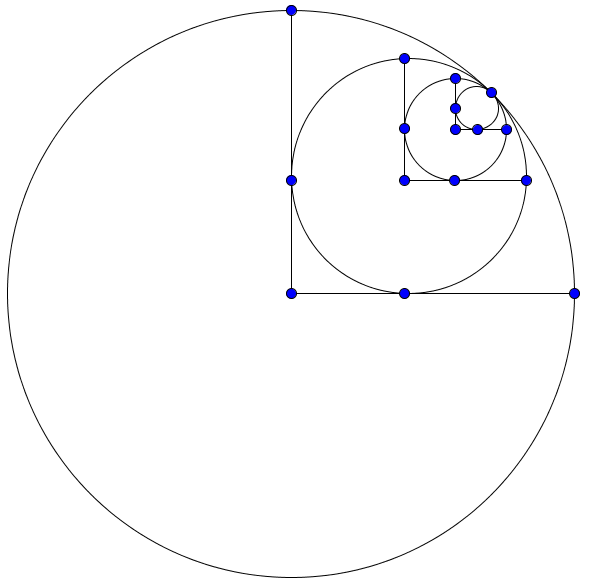# Inspired by Ethan

Geometry Level 5If the pattern of inscribed circles shown in the image above is repeated infinitely, each new circle is inscribed within a quadrant of the larger circle and the radius of the largest circle is 10, then what is the area of all the circles?

The area of all the circles can be expressed as $a\pi (\sqrt b + c)$, where $a,b$ and $c$ are integers with $b$ square-free. Find $a+b+c$.

×

Problem Loading...

Note Loading...

Set Loading...